• NTT 多项式乘法前言前置知识多项式的表示单位根离散傅里叶变换(DFT)快速傅里叶变换(FFT)离散傅里叶逆变换(IDFT)快速傅里叶逆变换FTT实现优化NTT 前言 FFT，快速傅里叶变换;NTT，快速数论变换，其实是一个东西在不同...

FFT&NTT 多项式乘法

前言

FFT，快速傅里叶变换;NTT，快速数论变换，其实是一个东西在不同的域上的不同表现形式。本博客只是简单地总结一下，提一些其它博客没有注意的地方。

推荐学习资料：

OI Wiki-FFT

OI Wiki-NTT

NTT与多项式全家桶——command block

前置知识

多项式的表示

1. 系数表示。要表示一个度为 n n 的多项式，只要 n + 1 n+1 个数表达 x i ( 0 ≤ i ≤ n ) x^i(0\le i\le n) 项的系数即可。
2. 点值表示。只要 n + 1 n+1 个横坐标不同的点，也可以表示这个多项式。这是因为代入 n + 1 n+1 个点，可以得到 n + 1 n+1 个方程，把 n + 1 n+1 个系数看成未知数，就变成了 小学二年级学过的 多元一次方程组啦。值得注意的是，这里的点的横纵坐标不必为实数，比如我们 FFT 用到的就是横纵坐标都为复数的点。

如何快速计算乘法？如果是系数表示，我们需要 O ( n 2 ) \mathcal O(n^2) 的复杂度。但是点值在这方面异常优秀，只要 O ( n ) \mathcal O(n) 即可。

单位根

我们把复平面上单位圆 n n 等分（以 ( 1 , 0 ) (1,0) 作为等分的第一个点），会得到 n n 个点。把这 n n 个点对应的复数叫做 n n 次单位根。记作 w n j w_n^j ，其中 0 ≤ j < n 0\le j<n w n j w_n^j 的模为 1，辐角为 j 2 π \dfrac{j}{2\pi} 。于是有

w n j = exp ⁡ ( i 2 π j n ) = cos ⁡ 2 π j n + i sin ⁡ 2 π j n w_n^j=\exp(i\frac{2\pi j}{n})=\cos\dfrac {2\pi j}{n}+i\sin\dfrac {2\pi j}n

单位根有优美的性质：

1. w n j = w n j + k n , k ∈ Z w_n^j=w_n^{j+kn},k\in\Z
2. w n j = w 2 n 2 j w_n^j=w_{2n}^{2j}
3. w 2 n j + n = − w 2 n j w_{2n}^{j+n}=-w_{2n}^j

这些性质是我们利用 FFT 快速计算的基石。

离散傅里叶变换(DFT)

离散傅里叶变换，就是将系数表示变为点值表示（即“求值”）。这个大家都会， O ( n 2 ) \mathcal O(n^2) 暴力代入啊。可惜太慢了。

快速傅里叶变换(FFT)

快速傅里叶变换利用 单位根 的性质，分治 的方法，在 O ( n log ⁡ n ) \mathcal O(n\log n) 的时间内将一个度数为 n n 的多项式由系数表示变为点值表示。它是 DFT的升级版。

比如一个度数为 n − 1 n-1 （这里假设 n n 是2的整数次幂）的多项式

f ( x ) = a 0 + a 1 x + a 2 x 2 + ⋯ + a n − 1 x n − 1 f(x)=a_0+a_1x+a_2x^2+\cdots+a_{n-1}x^{n-1}

我们分一下奇偶。

f ( x ) = ( a 0 + a 2 x 2 + ⋯ + a n − 2 x n − 2 ) + ( a 1 x + a 3 x 3 + ⋯ + a n − 1 x n − 1 ) f(x)=(a_0+a_2x^2+\cdots+a_{n-2}x^{n-2})+(a_1x+a_3x^3+\cdots+a_{n-1}x^{n-1})

= ( a 0 + a 2 x 2 + ⋯ + a n − 2 x n − 2 ) + x ( a 1 + a 3 x 2 + ⋯ + a n − 1 x n − 2 ) =(a_0+a_2x^2+\cdots+a_{n-2}x^{n-2})+x(a_1+a_3x^2+\cdots+a_{n-1}x^{n-2})

我们记 f 1 ( x ) = a 0 + a 2 x + ⋯ + a n − 2 x n / 2 − 1 f_1(x)=a_0+a_2x+\cdots+a_{n-2}x^{n/2-1} f 2 ( x ) = a 1 + a 3 x + ⋯ + a n − 1 x n / 2 − 1 f_2(x)=a_1+a_3x+\cdots+a_{n-1}x^{n/2-1}

则有 f ( x ) = f 1 ( x 2 ) + x f 2 ( x 2 ) f(x)=f_1(x^2)+xf_2(x^2)

为了快速计算，我们带入单位根。

1. 先带入个 w n k ( k < n / 2 ) w_n^k(k<n/2)

f ( w n k ) = f 1 ( w n / 2 k ) + w n k f 2 ( w n / 2 k ) f(w_n^k)=f_1(w_{n/2}^{k})+w_n^kf_2(w_{n/2}^k)

1. 再带入个 w n k + n / 2 = − w n k ( k < n / 2 ) w_n^{k+n/2}=-w_n^k(k<n/2)

f ( w n k + n / 2 ) = f 1 ( w n / 2 k ) − w n k f 2 ( w n / 2 k ) f(w_n^{k+n/2})=f_1(w_{n/2}^k)-w_n^kf_2(w^k_{n/2})

我们发现，两次代入有惊人的相似性

于是可以分治计算了。每一层 n n 的规模规模减半，显然复杂度是 O ( n log ⁡ n ) O(n\log n)

代码就不找了，随便一篇博客都有。

离散傅里叶逆变换(IDFT)

离散傅里叶逆变换，就是将点值表示变为系数表示（即“插值”）。怎么做？高斯消元是 O ( n 3 ) O(n^3) 的。似乎不怎么好做。

快速傅里叶逆变换

还是请回我们的单位根吧，看看怎么做。。。

结论：把点值（ f ( w n k ) f(w_n^k) ）当成系数，将 DFT中乘的那个 w n k w_n^k 换成 − w n k -w_n^k 求点值，最后再都除以 n n ，就是原多项式的系数啦。

证明去找别的博客啦。

FTT实现

这里加上了 “蝴蝶效应” 变成了迭代写法，可以大幅度减小常数。

模板：

#include<cstdio>
#include<cstring>
#include<algorithm>
#include<cmath>
using namespace std;
typedef long long ll;
typedef double db;
char In[1 << 20], *ss = In, *tt = In;
#define getchar() (ss == tt && (tt = (ss = In) + fread(In, 1, 1 << 20, stdin), ss == tt) ? EOF : *ss++)
ll x = 0, f = 1; char ch = getchar();
for(; ch < '0' || ch > '9'; ch = getchar()) if(ch == '-') f = -1;
for(; ch >= '0' && ch <= '9'; ch = getchar()) x = x * 10 + int(ch - '0');
return x * f;
}
const int MAXN = 5e6 + 5;
const db Pi = acos(-1.0);
struct cp {db x, y;}f[MAXN], g[MAXN];
cp operator + (const cp& a, const cp& b) {return (cp){a.x+b.x, a.y+b.y};}
cp operator - (const cp& a, const cp& b) {return (cp){a.x-b.x, a.y-b.y};}
cp operator * (const cp& a, const cp& b) {return (cp){a.x*b.x - a.y*b.y, a.x*b.y + a.y*b.x};}
int n, m, d, id[MAXN];
void fft(cp* f, int fl) {
for(int i = 0; i < d; i++) if(i < id[i]) swap(f[i], f[id[i]]);
for(int l = 2, hl = 1; l <= d; l <<= 1, hl <<= 1) {
//这是在枚举哪一层，这里的 l 就是推柿子时的 n
cp w0 = (cp){cos(2*Pi / l), fl * sin(2*Pi / l)};
for(int i = 0; i < d; i += l) {//i是每次迭代的段头
cp w = (cp){1, 0};
for(int j = i; j < i+hl; j++, w = w * w0) {//j则是控制推柿子时的 k
cp tt = w * f[j+hl];
f[j+hl] = f[j] - tt;
f[j] = f[j] + tt;
}
}
}
if(fl == -1) {//idft还得除以个 d（懒得写数乘，就直接这样写了）
for(int i = 0; i < d; i++) f[i].x /= d, f[i].y /= d;
}
}
int main() {
for(int i = 0; i <= n; i++) f[i].x = read();
for(int i = 0; i <= m; i++) g[i].x = read();
for(d = 1; d <= n+m; d <<= 1);
for(int i = 0; i <= d; i++)
id[i] = (id[i >> 1] >> 1) | ((i & 1) ? (d >> 1) : 0);
fft(f, 1); fft(g, 1);
for(int i = 0; i < d; i++) f[i] = f[i] * g[i];
fft(f, -1);
for(int i = 0; i <= n+m; i++) printf("%d ", int(f[i].x + 0.5));
return 0;
}

请全文背诵

注意事项：

1. 数组空间请注意，要开到 n + m n+m 的至少两倍。
2. 请注意精度误差，如果 f f g g 的数量级差很多，不妨先数乘到同一数量级再做。

优化

我们可以利用一下 f , g f,g 系数的虚部为零的特点，“三次变两次”。

我们构造一个系数为复数的多项式 h ( x ) = f ( x ) + i g ( x ) h(x)=f(x)+ig(x)

那么 h 2 ( x ) = f 2 ( x ) − g 2 ( x ) + i ⋅ ( 2 f ( x ) g ( x ) ) h^2(x)=f^2(x)-g^2(x)+i\cdot(2f(x)g(x))

于是我们只要构造 h h ，让其平方即可。只要一次DFT和一次IDFT。

#include<cstdio>
#include<cstring>
#include<algorithm>
#include<cmath>
using namespace std;
typedef long long ll;
typedef double db;
char In[1 << 20], *ss = In, *tt = In;
#define getchar() (ss == tt && (tt = (ss = In) + fread(In, 1, 1 << 20, stdin), ss == tt) ? EOF : *ss++)
ll x = 0, f = 1; char ch = getchar();
for(; ch < '0' || ch > '9'; ch = getchar()) if(ch == '-') f = -1;
for(; ch >= '0' && ch <= '9'; ch = getchar()) x = x * 10 + int(ch - '0');
return x * f;
}
const int MAXN = 5e6 + 5;
const db Pi = acos(-1.0);
struct cp{db x, y;}f[MAXN];
cp operator + (const cp& a, const cp& b) {return (cp){a.x+b.x, a.y+b.y};}
cp operator - (const cp& a, const cp& b) {return (cp){a.x-b.x, a.y-b.y};}
cp operator * (const cp& a, const cp& b) {return (cp){a.x*b.x - a.y*b.y, a.x*b.y + a.y * b.x};}
int n, m, d, id[MAXN];
void fft(cp* f, int fl) {
for(int i = 0; i < d; i++) if(i < id[i]) swap(f[i], f[id[i]]);
for(int l = 2, hl = 1; l <= d; l <<= 1, hl <<= 1) {
cp w0 = (cp){cos(2*Pi / l), fl * sin(2*Pi / l)};
for(int i = 0; i < d; i += l) {
cp w = (cp){1, 0};
for(int j = i; j < i + hl; j++, w = w * w0) {
cp tt = w * f[j+hl];
f[j+hl] = f[j] - tt;
f[j] = f[j] + tt;
}
}
}
if(fl == -1) {
for(int i = 0; i < d; i++) f[i].x /= d, f[i].y /= d;
}
}
int main() {
for(int i = 0; i <= n; i++) f[i].x = read();
for(int i = 0; i <= m; i++) f[i].y = read();
for(d = 1; d <= n+m; d <<= 1);
for(int i = 0; i < d; i++) id[i] = (id[i >> 1] >> 1) | ((i & 1) ? (d >> 1) : 0);
fft(f, 1);
for(int i = 0; i < d; i++) f[i] = f[i] * f[i];
fft(f, -1);
for(int i = 0; i < d; i++) f[i].y /= 2;
for(int i = 0; i <= n+m; i++) printf("%d ", int(f[i].y + 0.5));
return 0;
}

NTT

我们之前都是在复数域内搞东西，但如果在模意义下，系数可能较大，题目要求取模。这时 FFT 就无用武之地了。幸运的是，我们有完美的替代品：NTT。

这里需要用到 原根

我们可以把 g j ( p − 1 ) / n g^{j(p-1)/n} 当成 n n 次单位根 w n j w_n^j

1. w n j = w n j + k n , k ∈ Z w_n^j=w_n^{j+kn},k\in\Z
2. w n j = w 2 n 2 j w_n^j=w_{2n}^{2j}
3. w 2 n j + n = − w 2 n j w_{2n}^{j+n}=-w_{2n}^j

我们仍然有这些性质成立。

于是我们可以把它直接看成是单位根，FFT就变成NTT了。所有运算在模意义下完成。

常见的模数和它的原根

主要记住：

998244353，原根是 3

1004535809，原根是 3

#include<cstdio>
#include<cstring>
#include<algorithm>
using namespace std;
typedef long long ll;
char In[1 << 20], *ss = In, *tt = In;
#define getchar() (ss == tt && (tt = (ss = In) + fread(In, 1, 1 << 20, stdin), ss == tt) ? EOF : *ss++)
ll x = 0, f = 1; char ch = getchar();
for(; ch < '0' || ch > '9'; ch = getchar()) if(ch == '-') f = -1;
for(; ch >= '0' && ch <= '9'; ch = getchar()) x = x * 10 + int(ch - '0');
return x * f;
}
const int MAXN = 5e6 + 5;
const int P = 998244353, G = 3, invG = 332748118;
ll pls(ll a, ll b) {return a + b < P ? a + b : a + b - P;}
ll mns(ll a, ll b) {return a < b ? a + P - b : a - b;}
ll mul(ll a, ll b) {return a * b % P;}
int n, m, d, id[MAXN];
ll f[MAXN], g[MAXN];
ll qpow(ll a, ll n) {
ll ret = 1;
for(; n; n >>= 1, a = mul(a, a))
if(n & 1) ret = mul(ret, a);
return ret;
}
void NTT(ll* f, int n, int fl) {
for(int i = 0; i < n; i++) if(i < id[i]) swap(f[i], f[id[i]]);
for(int l = 2, hl = 1; l <= n; l <<= 1, hl <<= 1) {
ll g0 = qpow(fl == 1 ? G : invG, (P-1) / l);
for(int i = 0; i < n; i += l) {
ll gn = 1;
for(int j = i; j < i + hl; j++, gn = mul(gn, g0)) {
ll tt = mul(f[j+hl], gn);
f[j+hl] = mns(f[j], tt);
f[j] = pls(f[j], tt);
}
}
}
if(fl == -1) {
ll invn = qpow(n, P-2);
for(int i = 0; i < n; i++) f[i] = mul(f[i], invn);
}
}
int main() {
for(int i = 0; i <= n; i++) f[i] = read();
for(int i = 0; i <= m; i++) g[i] = read();
for(d = 1; d <= n+m; d <<= 1);
for(int i = 0; i < d; i++) id[i] = (id[i >> 1] >> 1) | ((i & 1) ? (d >> 1) : 0);
NTT(f, d, 1); NTT(g, d, 1);
for(int i = 0; i < d; i++) f[i] = mul(f[i], g[i]);
NTT(f, d, -1);
for(int i = 0; i <= n+m; i++) printf("%lld ", f[i]);
printf("\n");
return 0;
}

多项式乘法封装

#define clr(f, s, e) memset(f+(s), 0x00, sizeof(int) * ((e) - (s)))
#define cpy(f, g, n) memcpy(g, f, sizeof(int) * (n))
const int MAXN = (1 << 18) + 1, bas = 1 << 18, P = 998244353, G = 3, invG = 332748118;
int pls(int a, int b) {return a + b < P ? a + b : a + b - P;}
int mns(int a, int b) {return a < b ? a + P - b : a - b;}
int mul(int a, int b) {return 1ll * a * b % P;}
int qpow(int a, int n) {int ret = 1; for(; n; n >>= 1, a = mul(a, a)) if(n & 1) ret = mul(ret, a); return ret;}
int tf, tr[MAXN], _g[MAXN], inv[MAXN];
void init() {
inv = 1; for(int i = 2; i < MAXN; i++) inv[i] = mul(P - P / i, inv[P % i]);
for(int i = 0; i < bas; i++) {
_g[i] = qpow(G, (P-1) / bas * i);
_g[i] = qpow(invG, (P-1) / bas * i);
}
}
int getlim(int n) {
int lim = 1; for(; lim < n + n; lim <<= 1);
return lim;
}
void tpre(int lim) {
if(lim == tf) return ;
tf = lim; for(int i = 0; i < lim; i++) tr[i] = (tr[i >> 1] >> 1) | ((i & 1) ? (lim >> 1) : 0);
}
void NTT(int* f, int lim, int fl) {
tpre(lim); for(int i = 0; i < lim; i++) if(i < tr[i]) swap(f[i], f[tr[i]]);
for(int l = 2, k = 1; l <= lim; l <<= 1, k <<= 1)
for(int i = 0; i < lim; i += l)
for(int j = i; j < i+k; j++) {
ll tt = mul(f[j+k], _g[fl][(j-i) * (bas / l)]);
f[j+k] = mns(f[j], tt);
f[j] = pls(f[j], tt);
}
if(!fl)
for(int i = 0; i < lim; i++) f[i] = mul(f[i], inv[lim]);
}
void Mul(int* f, int* g, int* h, int n) {
static int a[MAXN], b[MAXN];
int lim = getlim(n);
cpy(f, a, n); clr(a, n, lim);
cpy(g, b, n); clr(b, n, lim);
NTT(a, lim, 1); NTT(b, lim, 1);
for(int i = 0; i < lim; i++) h[i] = mul(a[i], b[i]);
NTT(h, lim, 0); clr(h, n, lim);
}

任意模数多项式乘法

P4245 【模板】任意模数多项式乘法

给 2 个多项式 F ( x ) , G ( x ) F(x),G(x) ，求 F ( x ) G ( x ) F(x)G(x) 。系数对 p p 取模，不保证 p p 是 NTT 模数。

也就是MTT，使用 4 次 FFT 完成任意模数的多项式乘法。

K = 2 15 K=2^{15} ，我们把多项式每项系数分为两部分（高低位）。
F ( x ) = K ⋅ F 1 ( x ) + F 0 ( x ) G ( x ) = K ⋅ G 1 ( x ) + G 0 ( x ) ∴ F ( x ) G ( x ) = K 2 ⋅ F 1 ( x ) G 1 ( x ) + K ⋅ [ F 1 ( x ) G 0 ( x ) + F 0 ( x ) G 1 ( x ) ] + F 0 ( x ) G 0 ( x ) F(x)=K\cdot F_1(x)+F_0(x) \\ G(x)=K\cdot G_1(x)+G_0(x) \\ \therefore F(x)G(x)=K^2\cdot F_1(x)G_1(x)+K\cdot [F_1(x)G_0(x)+F_0(x)G_1(x)]+F_0(x)G_0(x)
如何快速得到这四个多项式的点值表示？

构造
P ( x ) = F 0 ( x ) + i G 0 ( x ) Q ( x ) = F 0 ( x ) − i G 0 ( x ) P(x)=F_0(x)+iG_0(x) \\ Q(x)=F_0(x)-iG_0(x)
我们惊奇地发现：
D F T ( P ) [ j ] = P ( w n j ) = F 0 ( w n j ) + i G 0 ( w n j ) = ∑ k = 0 n − 1 F 0 [ k ] w n k j + i ∑ k = 0 n − 1 G 0 [ k ] w n k j = ∑ k = 0 n − 1 ( F 0 [ k ] + i G 0 [ k ] ) ( cos ⁡ ( 2 π k j n ) + i sin ⁡ ( 2 π k j n ) ) = ∑ k = 0 n − 1 ( F 0 [ k ] cos ⁡ ( 2 π k j n ) − G 0 [ k ] sin ⁡ ( 2 π k j n ) ) + i ∑ k = 0 n − 1 ( F 0 [ k ] sin ⁡ ( 2 π k j n ) + G 0 [ k ] sin ⁡ ( 2 π k j n ) ) \mathrm{DFT}(P)[j]=P(w_n^j)=F_0(w_n^j)+iG_0(w_n^j) \\ =\sum_{k=0}^{n-1}F_0[k]w_n^{kj}+i\sum_{k=0}^{n-1}G_0[k]w_n^{kj} \\ =\sum_{k=0}^{n-1}(F_0[k]+iG_0[k])(\cos(\dfrac {2\pi kj}{n})+i\sin(\dfrac{2\pi kj}n)) \\ =\sum_{k=0}^{n-1}(F_0[k]\cos(\dfrac{2\pi kj}n)-G_0[k]\sin(\dfrac {2\pi kj}n))+\\ i\sum_{k=0}^{n-1}(F_0[k]\sin(\dfrac {2\pi kj}n)+G_0[k]\sin(\dfrac {2\pi kj}n))

同理
D F T ( Q ) [ n − j ] = P ( w n − j ) = F 0 ( w n − j ) − i G 0 ( w n − j ) = ∑ k = 0 n − 1 F 0 [ k ] w n − k j − i ∑ k = 0 n − 1 G 0 [ k ] w n − k j = ∑ k = 0 n − 1 ( F 0 [ k ] − i G 0 [ k ] ) ( cos ⁡ ( 2 π k j n ) − i sin ⁡ ( 2 π k j n ) ) = ∑ k = 0 n − 1 ( F 0 [ k ] cos ⁡ ( 2 π k j n ) − G 0 [ k ] sin ⁡ ( 2 π k j n ) ) + i ∑ k = 0 n − 1 ( F 0 [ k ] sin ⁡ ( 2 π k j n ) + G 0 [ k ] sin ⁡ ( 2 π k j n ) ) \mathrm{DFT}(Q)[n-j]=P(w_n^{-j})=F_0(w_n^{-j})-iG_0(w_n^{-j}) \\ =\sum_{k=0}^{n-1}F_0[k]w_n^{-kj}-i\sum_{k=0}^{n-1}G_0[k]w_n^{-kj} \\ =\sum_{k=0}^{n-1}(F_0[k]-iG_0[k])(\cos(\dfrac {2\pi kj}{n})-i\sin(\dfrac{2\pi kj}n)) \\ =\sum_{k=0}^{n-1}(F_0[k]\cos(\dfrac{2\pi kj}n)-G_0[k]\sin(\dfrac {2\pi kj}n))+\\ i\sum_{k=0}^{n-1}(F_0[k]\sin(\dfrac {2\pi kj}n)+G_0[k]\sin(\dfrac {2\pi kj}n))
P P 的第 j j 项点值与 Q Q 的第 n − j n-j 项点值共轭。

于是我们可以使用 1 次 FFT 得到 P ( x ) P(x) Q ( x ) Q(x) 的点值，再解方程就可得到 F 0 ( x ) F_0(x) G 0 ( x ) G_0(x) 的点值。

同样地可得到 F 1 ( x ) , G 1 ( x ) F_1(x),G_1(x) ，使用了 2 次FFT。

然后考虑怎么求解 回系数。

构造
P ( x ) = F 1 ( x ) G 1 ( x ) + i ( F 1 ( x ) G 0 ( x ) + F 0 ( x ) G 1 ( x ) ) Q ( x ) = F 0 ( x ) G 0 ( x ) P(x)=F_1(x)G_1(x)+i(F_1(x)G_0(x)+F_0(x)G_1(x)) \\ Q(x)=F_0(x)G_0(x)
做两次 IDFT 即可。

#define clr(f, s, t) memset(f + (s), 0x00, sizeof(int) * ((t) - (s)))
#define cpy(f, g, n) memcpy(g, f, sizeof(int) * (n))
const int MAXN = (1 << 19) + 5, bas = 1 << 19;
const db PI = acos(-1.0);
int P;
int pls(int a, int b) {return a + b < P ? a + b : a + b - P;}
int mns(int a, int b) {return a < b ? a + P - b : a - b;}
int mul(int a, int b) {return 1ll * a * b % P;}
int qpow(int a, int n) {int ret = 1; for(; n; n >>= 1, a = mul(a, a)) if(n & 1) ret = mul(ret, a); return ret;}
struct cp {db x, y;};
cp operator + (const cp& a, const cp& b) {return (cp){a.x + b.x, a.y + b.y};}
cp operator - (const cp& a, const cp& b) {return (cp){a.x - b.x, a.y - b.y};}
cp operator * (const cp& a, const cp& b) {return (cp){a.x * b.x - a.y * b.y, a.x * b.y + a.y * b.x};}
cp operator * (const cp& a, const db& k) {return (cp){a.x * k, a.y * k};}
const cp I = (cp){0, 1};
cp _g[MAXN];
int tr[MAXN], tf;
void init() {
for(int i = 0; i < bas; i++) {
db a = cos(2 * PI * i / bas), b = sin(2 * PI * i / bas);
_g[i] = (cp){a, b};
_g[i] = (cp){a, -b};
}
}
int getlim(int n) {
int lim = 1; for(; lim < n + n; lim <<= 1);
return lim;
}
void tpre(int lim) {
if(tf == lim) return;
tf = lim; for(int i = 0; i < lim; i++) tr[i] = (tr[i >> 1] >> 1) | ((i & 1) ? (lim >> 1) : 0);
}
ll tran(db x) {return ((ll)(x > 0 ? x + .5 : x - .5) % P + P) % P;}
void FFT(cp* f, int lim, int fl) {
tpre(lim); for(int i = 0; i < lim; i++) if(i < tr[i]) swap(f[i], f[tr[i]]);
for(int l = 2, k = 1; l <= lim; l <<= 1, k <<= 1)
for(int i = 0; i < lim; i += l)
for(int j = i; j < i+k; j++) {
cp tt = f[j+k] * _g[fl][(j-i) * (bas / l)];
f[j+k] = f[j] - tt;
f[j] = f[j] + tt;
}
if(!fl) for(int i = 0; i < lim; i++) f[i].x /= lim, f[i].y /= lim;
}
void Mul(int* f, int* g, int* h, int n) {
static cp f0[MAXN], f1[MAXN], g0[MAXN], g1[MAXN];
int lim = getlim(n);
for(int i = 0; i < n; i++) f0[i].x = f[i] >> 15, f0[i].y = f[i] & 32767;
for(int i = 0; i < n; i++) g0[i].x = g[i] >> 15, g0[i].y = g[i] & 32767;
for(int i = n; i < lim; i++) f0[i] = (cp){0, 0};
for(int i = n; i < lim; i++) g0[i] = (cp){0, 0};
FFT(f0, lim, 1); FFT(g0, lim, 1);
for(int i = 0; i < lim; i++) {
f1[i] = f0[i ? lim - i : 0], f1[i].y *= -1;
g1[i] = g0[i ? lim - i : 0], g1[i].y *= -1;
}
for(int i = 0; i < lim; i++) {
cp a = (f0[i] + f1[i]) * 0.5;		//f0
cp b = (f1[i] - f0[i]) * 0.5 * I;	//f1
cp c = (g0[i] + g1[i]) * 0.5;		//g0
cp d = (g1[i] - g0[i]) * 0.5 * I;	//g1
f0[i] = a * c + I * (a * d + b * c);
g0[i] = b * d;
}
FFT(f0, lim, 0); FFT(g0, lim, 0);
for(int i = 0; i < n; i++)
h[i] = (1ll * tran(f0[i].x) * (1 << 30) + 1ll * tran(f0[i].y) * (1 << 15) % P + tran(g0[i].x)) % P;
clr(h, n, lim);
}

展开全文数学 多项式
• 在本文中，我们介绍了几种优化技术，以利用数论变换（NTT加速多项式乘法。... 在Spartan-6 FPGA上进行的实验结果表明，与高效实现技术水平相比，我们的多项式乘法器平均可实现2.04的加速，并消耗更少的硬件资源。
• 在本文中，我们介绍了几种优化技术，以利用数论变换（NTT）构建有效的多项式乘法器。 我们提出了一种优化NTT和反向NTT的位反向操作的技术。 通过其他优化，我们的多项式乘法器将所需的时钟周期从（8n + 1.5n lg n）...研究论文
• 来源：AI科技评论作者：Ryan Hamerly编译：陈彩娴近日，来自日本 NTT 研究所的高级科学家 Ryan Hamerly 在 IEEE Spectrum 上发表了一篇文章（“The...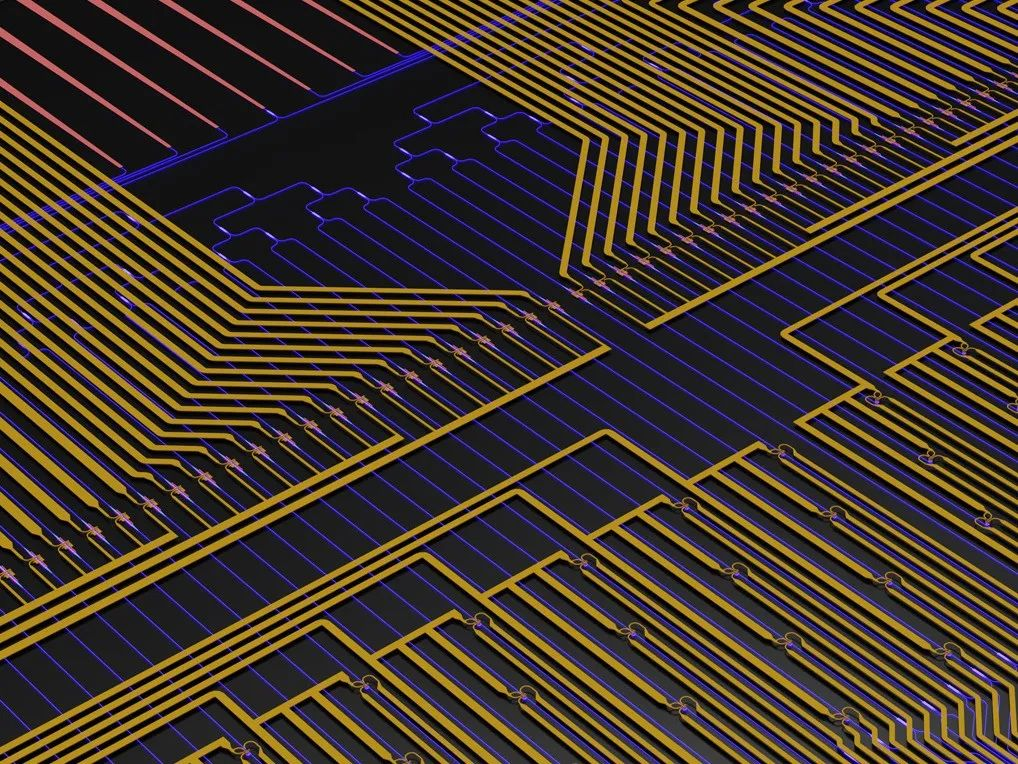来源：AI科技评论

作者：Ryan Hamerly

编译：陈彩娴

近日，来自日本 NTT 研究所的高级科学家 Ryan Hamerly 在 IEEE Spectrum 上发表了一篇文章（“The Future of Deep Learning Is Photonic”），谈论了光学计算在未来的强大潜力。他解释了为何光学计算会降低神经网络计算的能耗，以及光子设备取代电子设备的可能。

Ryan Hamerly 的本科就读于加州理工学院物理专业，2016年从斯坦福大学获得应用物理学博士学位。博士毕业后，他先是在日本 NTT 研究所担任博士后研究员，与日本知名研究员 Yoshihisa Yamamoto、Shoko Utsunomiya 研究光学与量子计算，一年后又到 MIT 做了两年博士后，博士后导师为 Dirk Englund 教授。图注：Ryan Hamerly

我们注意到，之前获得麻省理工科技评论“35岁以下科技创新35人”的中国青年科学家沈亦晨也在光子研究上有所成就。沈亦晨的博士毕业于 MIT，在2017年与 Nicholas Harris 发表了一篇如今谷歌学术引用接近1000的论文（“Deep learning with coherent nanophotonic circuits”），谈到将光学应用于机器学习任务，比如语音和图像识别。图注：沈亦晨

在2017年的工作中，沈亦晨也曾提出一个开创性的想法，即用光子替代电子来进行神经网络计算。同年，他在创立了Lightelligence公司，一年半后开发出了世界上第一款光子芯片原型板卡，初步验证了光子的潜力。

以下，AI科技评论对 Ryan Hamerly 一文作了不改原理的整理：

1

研究背景

近年来，计算机技术被应用到许多原先需要依靠人类感官的任务中，会识别图像中的物体、转录语音、跨语言翻译、诊断疾病、玩复杂的游戏和驾驶汽车等等。

直接促成这些惊人发展的技术被称为“深度学习”。深度学习指的是一种被称为人工神经网络的数学模型。深度学习是机器学习的一个子领域，而机器学习是计算机科学下一个基于复杂模型与数据拟合的的分支。

虽然机器学习已经发展了很长时间，但深度学习是近年来才崛起，主要是因为计算资源增加、变得广泛可用，同时可以轻松收集用于训练神经网络的大量数据。算力的增长加快，但深度学习的计算需求增长得更快。这促使了工程师开发专门针对深度学习的电子硬件加速器，谷歌的张量处理单元 (TPU) 就是一个很好的例子。

在这里，我将描述一种解决该问题的新方法，就是使用光学处理器来执行神经网络计算，用光子替代电子。要了解光学如何解决神经网络的计算问题之前，我们首先要了解目前计算机如何进行神经网络的计算。

2

电子难以满足神经网络的计算需求

在绝大多数情况下，人工神经元是由在数字电子计算机上运行的特殊软件构建而成。这个软件会为特定的神经元输送多个输入和一个输出。每个神经元的状态都取决于其输入的加权和，这个过程会使用到一个非线性函数（称为“激活函数”）。这个神经元的输出，也就是“结果”，又会成为其他神经元的输入。

为了提高计算效率，这些神经元会被一组一组地分到不同的层中，神经元只会与相邻层的神经元连接。这样做的好处是可以使用线性代数的某些数学技巧来加快计算的速度。

这些线性代数计算是深度学习中对计算要求最高的部分，而且随着神经网络规模的增长，它们对计算的要求也会增长。训练（确定对每个神经元的输入应用哪个权重的过程）和推理（当神经网络给出想要的结果时）步骤也是同样的原理。

这些神秘的线性代数计算是怎样的？它们其实没有那么复杂。它们涉及对矩阵的运算，而矩阵只是数字的矩形数组。

这是个好消息，因为现代计算机硬件已经针对矩阵运算进行了很好的优化。在深度学习兴起很久之前，矩阵运算就已经是高性能计算的重要基础。用于深度学习的相关矩阵计算可以归结为大量的乘法和累加运算，就是将成对的数字相乘并将它们的乘积相加。

这些年来，深度学习需要越来越多的乘法累加运算。比如 LeNet，这是一个开创性的深度神经网络，主要用于图像分类。1998 年，它被证明在识别手写字母和数字方面优于其他机器技术。到了 2012 年，神经网络 AlexNet 出现，能够识别图像中数千种不同类型的物体，但它的乘法累加运算却是 LeNet 的 1600 倍。

从 LeNet 的雏形成果到 AlexNet，神经网络对计算性能的需求提高了近 11 倍。在这 14 年里，摩尔定律促进了大部分算力的增长，但目前的挑战是难以再保持过去的增长趋势，因为摩尔定律已经失去发展的动力。这时候，要提高算力，通常的解决方案是在一个问题上投入更多的计算资源，以及时间、金钱和精力。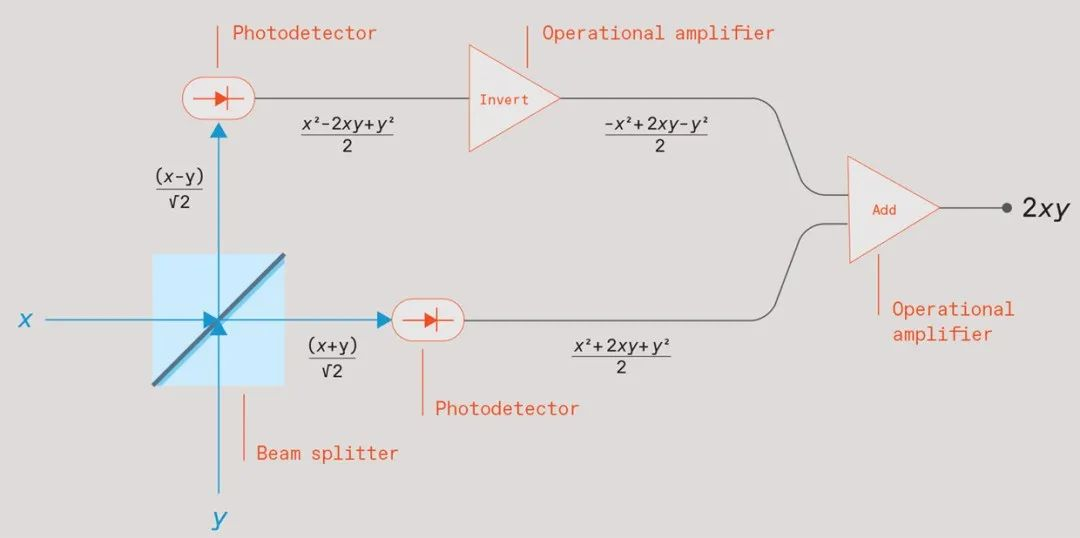图注：两束光线撞上一个光束分束器（蓝色方块），光束的强度与它们要相乘的数字 x 与 y 相当。离开分束器的光束照在光电探测器（椭圆形）上，提供与光强度平方成正比的电信号。将一个光电探测器信号调反，并将其与另一个信号相加，就会产生一个与两个输入的乘积成正比的信号。

所以，训练当今的大型神经网络通常会带来显著的环境问题。例如，2019 年的一项研究（“Energy and Policy Considerations for Deep Learning in NLP”）发现，训练一个特定的深度神经网络来进行自然语言处理所产生的二氧化碳排放量是汽车在整个生命周期内的驾驶所产生的二氧化碳排放量的五倍。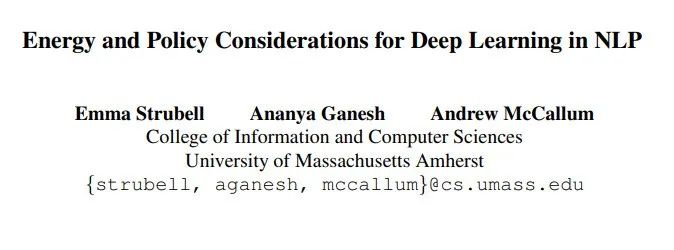论文地址：https://arxiv.org/pdf/1906.02243.pdf

3

光学应运而生

可以肯定的是，数字电子计算机的进步促进了深度学习的蓬勃发展，但这并不意味电子计算机是执行神经网络计算的唯一机器。数十年前，当数字计算机还相对原始时，一些工程师是使用模拟计算机来解决困难的计算。随着数字电子技术的进步，那些模拟计算机被淘汰了。

但现在也许是再次使用模拟计算机的好时机，尤其是当模拟计算可以通过光学的方式来完成时。

我们知道，光纤可以支持比电线高得多的数据速率。这也是为什么从 1970 年代后期开始，所有长途通信线路都采用光纤的原因。从那时起，光数据线路取代了铜线，已实现越来越短的跨度，一路延伸到数据中心的机架到机架通信。光数据通信速度更快，能耗也更低。光学计算也有同样的优势。

但数据传播与用数据进行计算有很大的区别。这也是模拟光学所遇到的障碍。传统的计算机是基于晶体管，而晶体管是高度非线性的电路元件——这意味着它们的输出不仅与输入成正比，至少在用于计算时是这样。非线性决定了晶体管的开关，使得它们可以被塑造成逻辑门电路。这种切换很容易用电子设备来完成，所以电子设备的非线性十分重要。但光子遵循的是麦克斯韦方程，是线性的，这就意味着光学设备的输出通常与其输入成正比。

在这里，一个诀窍是利用光学设备的线性来做深度学习最依赖的部分——线性代数。

为了解释如何做到这一点，我将在这里描述这样一个光子设备：当它与一些简单的模拟电子设备耦合时，它可以将两个矩阵相乘。这种乘法将一个矩阵的行与另一个矩阵的列组合在一起。更准确地说，它将这些行和列中的数对相乘，并将它们的乘积加在一起——也就是我之前描述的乘法和累加运算。我和我在 MIT 的同事在 2019 年发表了一篇文章（“Large-Scale Optical Neural Networks Based on Photoelectric Multiplication”），解释了为什么可以光子设备做到这一点。我们正在努力构建这样一个光学矩阵乘法器。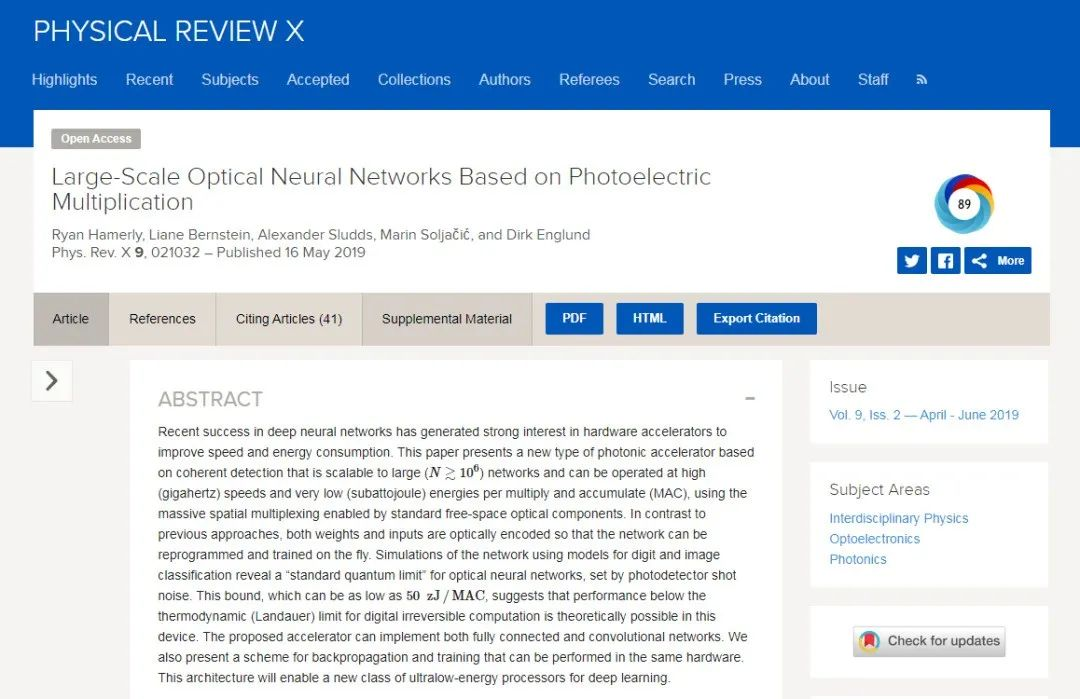论文地址：https://journals.aps.org/prx/abstract/10.1103/PhysRevX.9.021032

在这个光子设备中，最基础的计算单元是一个被称为“分束器”的光学元件。分束器的实际组装很复杂，但你可以把它想象成一个 45 度角的半镀银镜子。如果您从侧面向其发送一束光，分束器将允许一半光直接穿过它，而另一半则从有角度的镜子中反射，使其与入射光束成 90 度反弹。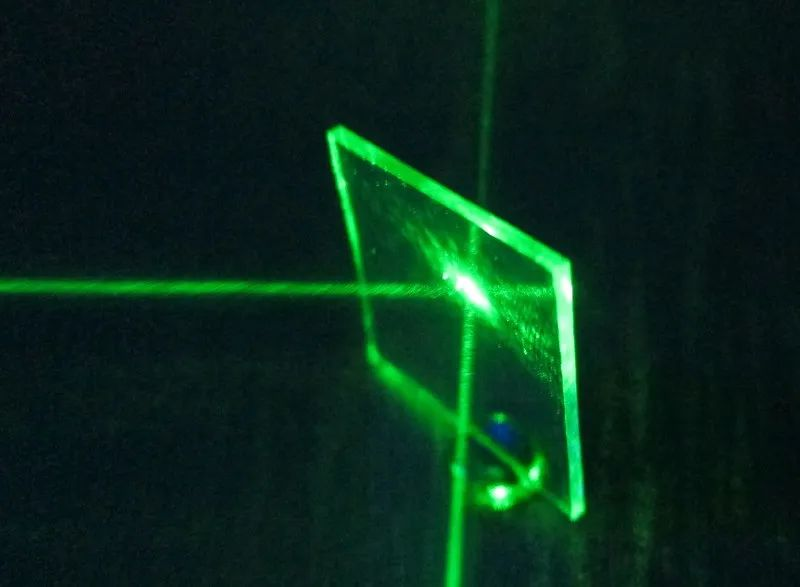图注：分束器

现在将第二束光垂直于第一束光照射到该分束器中，使其照射到成角度的镜子的另一侧。第二光束的一半将类似地以 90 度角透射和反射。两个输出光束将与第一个光束的两个输出成组合。所以这个分束器会有两个输入和两个输出。

要使用此设备进行矩阵乘法，你需要生成两个光束，且光束的电场强度与要相乘的两个数字成正比。我们将这些电场强度称为 x 和 y。将这两束光照射到分束器中，分束器会将这两束光合并在一起。这种特殊的分束器会产生两个输出，其电场值为 (x + y)/√2 和 (x − y)/√2。

除了分束器之外，这个模拟乘法器还需要两个简单的电子元件（两个光电探测器）来测量两个输出光束。不过，它们不测量这些光束的电场强度，而是测量光束的功率，该功率与其电场强度的平方成正比。

为什么这种联系很重要？要理解这一点，需要一些代数知识，但都是高中阶段的内容：当你对 (x + y)/√2 平方时，你会得到 (x2 + 2xy + y2)/2。当你平方 (x − y)/√2 时，你会得到 (x2 − 2xy + y2)/2。从前者中减去后者得到 2xy。

现在停下来思考这个简单数学的重要性。这意味着：如果你将一个数字编码为具有一定强度的光束，将另一个数字编码为另一种强度的光束，然后将它们通过这样的分束器发送，用光学探测器来测量两个输出，并在将它们相加之前抵消所产生的电信号之一，你就会得到一个与两个数字的乘积成正比的信号。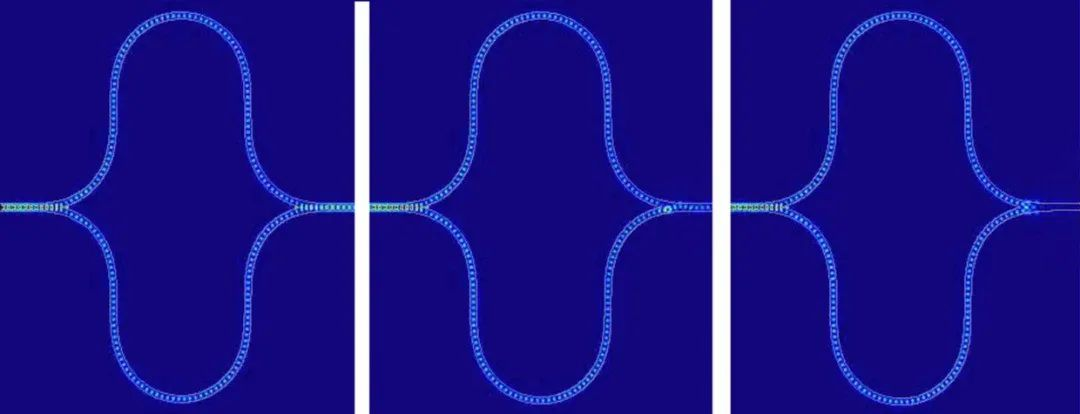图注：Lightmatter 的神经网络加速器中集成的 Mach-Zehnder 干涉仪模拟显示了三种不同的状态，其中在干涉仪的两个分支中传播的光经历了不同的相对相移。

我的描述听起来像是这些光束中的每一个光束都必须保持稳定。但事实上，你可以在两个输入光束中短暂地震动光并测量输出脉冲。更好的做法是，你可以将输出信号馈送到电容器中，只要震动持续，电容器就会积累电荷。然后，你可以在相同的时间段内再次脉冲输入，编码两个要相乘的新数字。它们的产品为电容器增加了一些电荷。你可以根据需要多次重复此过程，每一次都进行一次新的乘法累加运算。

以这种方式使用脉冲光可以让你飞速执行许多此类运算。其中，最耗能的部分是读取该电容器上的电压，这时需要一个模数转换器。但是你不必在每个脉冲后都这样做。你可以等到 N 个脉冲后结束后再统一读取。这意味着：该设备可以使用相同的能量来执行 N 次乘法累加运算，不管 N 是大是小。在这里，N 对应神经网络中每层的神经元数量，很容易达到数千个，所以用光子执行神经网络计算的能耗会很少。

有时候，你也可以在输入端节省能源，因为相同的值经常被用作多个神经元的输入。它不用多次将数字转换为光（每转换一次就会消耗一次能量），而是可以一次性转换所有，产生的光束可以被分成多个电流波段。通过这种方式，输入转换的能源成本可以在多次运算中分摊。

将一束光束分成多个波段需要透镜，但透镜很难安装在芯片上。因此，我们正在开发的以光学方式执行神经网络计算的设备很可能会是一种结合高集成光子芯片与单个光学元件的混合体。

4

其他实践案例

我在这里概述了我和我的同事一直在追求的策略，但从光学角度解决这个问题的方法不止一种。比如，另一个很有前景的方案是基于 Mach-Zehnder 干涉仪，它结合了两个分束器和两个全反射镜，也可以用光学方式运算矩阵乘法。两家有 MIT 学术背景的初创公司 Lightmatter 和 Lightelligence（沈亦晨创办） 正在开发基于 Mach-Zehnder 干涉仪的光学神经网络加速器。Lightmatter 已经制造出一个光学芯片原型，并有望在今年年底开始销售使用该芯片的光加速器板。

另一家使用光学进行计算的初创公司是 Optalysis。早在 1960 年代，光学计算的首批用途之一就是处理合成孔径雷达数据。但这有一个重大挑战，就是如何将傅立叶变换（一种数学运算）应用于要测量的数据上。当时的数字计算机一直在努力解决这些问题。即使是现在，将傅立叶变换应用于大规模数据也需要密集的计算。但是，傅立叶变换可以以光学的方式执行，只需要一个透镜（lens）——这也是多年来工程师处理合成孔径数据的方式。Optalysis 希望根据当前的需求改进这种方法，并广泛应用。

还有一家叫做 Luminous 的公司，源于普林斯顿大学，他们正致力于创建基于激光神经元的脉冲神经网络（spiking neural networks）。脉冲神经网络更像是模仿生物神经网络的运作方式，比如我们的大脑，能够使用低能量进行计算。Luminous 的硬件仍处于早期开发阶段，但结合脉冲和光学两种节能方法的潜力还是非常令人期待的！

5

光学的机遇与挑战

当然，目前仍有许多技术挑战需要克服。

一是要提高模拟光学计算的精度和动态范围，这方面还远不及数字电子设备所能达到的效果。这是因为这些光学处理器受到各种噪声源的影响，而且用于输入和输出数据的数模转换器和模数转换器精度有限。事实上，很难想象一个光学神经网络的运行精度超过 8 到 10 位。虽然存在 8 位电子深度学习硬件（比如 Google 的 TPU），但这个行业还需要更高的精度，尤其是用于神经网络训练时。

将光学元件集成到一块芯片上也很难。由于这些元件的尺寸为数十微米，它们无法像晶体管一样进行紧密封装，所以目标芯片的面积也会加大。2017年，来自 MIT 的团队（沈亦晨为一作）就针对这个问题发表了一篇工作（“Deep learning with coherent nanophotonic circuits”），谈到一种尺寸为 1.5 毫米的芯片。即使是最大的芯片也不会超过几平方厘米，这限制了可以用这种方式并行处理的矩阵的大小。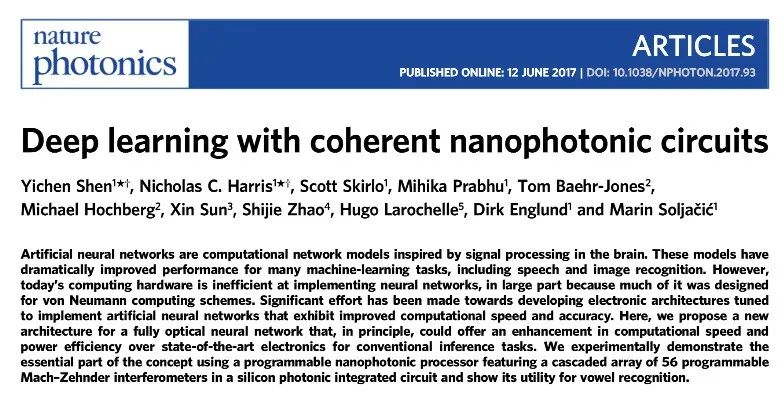论文地址：https://www.nature.com/articles/nphoton.2017.93.epdf

在计算机的架构方面，光学研究人员还有许多其他问题要解决。但可以肯定的是，至少在理论上，光学有希望将深度学习的发展加速几个数量级。

基于当前可用于各种组件（光调制器、检测器、放大器、模数转换器）的技术，我们有理由相信，神经网络计算的能源效率可以比当今的电子处理器提高 1,000 倍。如果用新兴的光学技术作出更激进的假设，神经网络计算的能源效率甚至可能提高一百万倍。而且，由于电子处理器的功率有限，这些能源效率的进步很可能会转化为相应的速度改进。

模拟光学计算中的许多概念已有数十年历史。有些概念的诞生甚至早于硅计算机。光学矩阵乘法、甚至光学神经网络的首次出现甚至可以追溯到1970年代。但当时这种方法并没有流行起来。但如今时代不同，光学计算的命运可能会有所改变，原因主要有三点：

• 首先，如今深度学习有真正的用途，而不仅仅是学术上的好奇；

• 其次，我们不能仅仅依靠摩尔定律来改进电子产品；

• 最后，我们有了前几代人没有的新技术：集成光子学。

这些因素表明，光神经网络将真正到来，而且，神经网络计算的未来可能是光子的。

原文链接：

未来智能实验室的主要工作包括：建立AI智能系统智商评测体系，开展世界人工智能智商评测；开展互联网（城市）大脑研究计划，构建互联网（城市）大脑技术和企业图谱，为提升企业，行业与城市的智能水平服务。每日推荐范围未来科技发展趋势的学习型文章。目前线上平台已收藏上千篇精华前沿科技文章和报告。

如果您对实验室的研究感兴趣，欢迎加入未来智能实验室线上平台。扫描以下二维码或点击本文左下角“阅读原文”展开全文神经网络 大数据 机器学习 人工智能
• 作者 | Ryan Hamerly编译 | 陈彩娴转自：AI科技评论近日，来自日本 NTT 研究所的高级科学家 Ryan Hamerly 在 IEEE Spectrum 上发表了一篇文章（...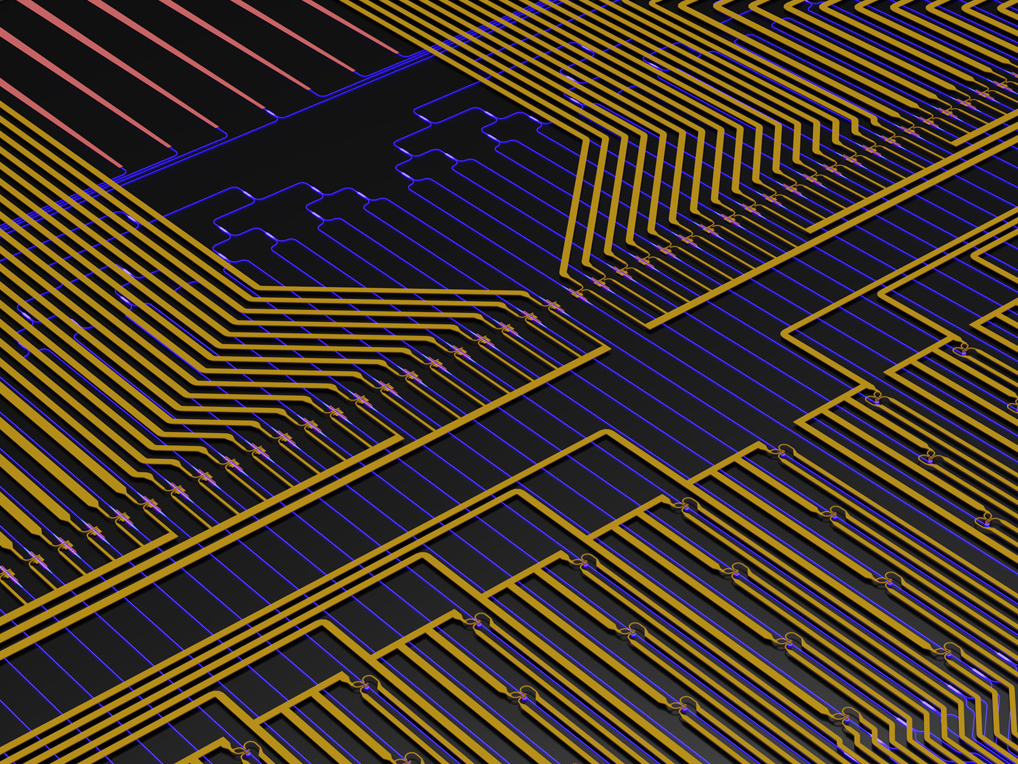作者 | Ryan Hamerly

编译 | 陈彩娴

转自：AI科技评论

近日，来自日本 NTT 研究所的高级科学家 Ryan Hamerly 在 IEEE Spectrum 上发表了一篇文章（“The Future of Deep Learning Is Photonic”），谈论了光学计算在未来的强大潜力。他解释了为何光学计算会降低神经网络计算的能耗，以及光子设备取代电子设备的可能。

Ryan Hamerly 的本科就读于加州理工学院物理专业，2016年从斯坦福大学获得应用物理学博士学位。博士毕业后，他先是在日本 NTT 研究所担任博士后研究员，与日本知名研究员 Yoshihisa Yamamoto、Shoko Utsunomiya 研究光学与量子计算，一年后又到 MIT 做了两年博士后，博士后导师为 Dirk Englund 教授。图注：Ryan Hamerly

我们注意到，之前获得麻省理工科技评论“35岁以下科技创新35人”的中国青年科学家沈亦晨也在光子研究上有所成就。沈亦晨的博士毕业于 MIT，在2017年与 Nicholas Harris 发表了一篇如今谷歌学术引用接近1000的论文（“Deep learning with coherent nanophotonic circuits”），谈到将光学应用于机器学习任务，比如语音和图像识别。图注：沈亦晨

在2017年的工作中，沈亦晨也曾提出一个开创性的想法，即用光子替代电子来进行神经网络计算。同年，他在创立了Lightelligence公司，一年半后开发出了世界上第一款光子芯片原型板卡，初步验证了光子的潜力。

1

研究背景

近年来，计算机技术被应用到许多原先需要依靠人类感官的任务中，会识别图像中的物体、转录语音、跨语言翻译、诊断疾病、玩复杂的游戏和驾驶汽车等等。

直接促成这些惊人发展的技术被称为“深度学习”。深度学习指的是一种被称为人工神经网络的数学模型。深度学习是机器学习的一个子领域，而机器学习是计算机科学下一个基于复杂模型与数据拟合的的分支。

虽然机器学习已经发展了很长时间，但深度学习是近年来才崛起，主要是因为计算资源增加、变得广泛可用，同时可以轻松收集用于训练神经网络的大量数据。算力的增长加快，但深度学习的计算需求增长得更快。这促使了工程师开发专门针对深度学习的电子硬件加速器，谷歌的张量处理单元 (TPU) 就是一个很好的例子。

在这里，我将描述一种解决该问题的新方法，就是使用光学处理器来执行神经网络计算，用光子替代电子。要了解光学如何解决神经网络的计算问题之前，我们首先要了解目前计算机如何进行神经网络的计算。

2

电子难以满足神经网络的计算需求

在绝大多数情况下，人工神经元是由在数字电子计算机上运行的特殊软件构建而成。这个软件会为特定的神经元输送多个输入和一个输出。每个神经元的状态都取决于其输入的加权和，这个过程会使用到一个非线性函数（称为“激活函数”）。这个神经元的输出，也就是“结果”，又会成为其他神经元的输入。

为了提高计算效率，这些神经元会被一组一组地分到不同的层中，神经元只会与相邻层的神经元连接。这样做的好处是可以使用线性代数的某些数学技巧来加快计算的速度。

这些线性代数计算是深度学习中对计算要求最高的部分，而且随着神经网络规模的增长，它们对计算的要求也会增长。训练（确定对每个神经元的输入应用哪个权重的过程）和推理（当神经网络给出想要的结果时）步骤也是同样的原理。

这些神秘的线性代数计算是怎样的？它们其实没有那么复杂。它们涉及对矩阵的运算，而矩阵只是数字的矩形数组。

这是个好消息，因为现代计算机硬件已经针对矩阵运算进行了很好的优化。在深度学习兴起很久之前，矩阵运算就已经是高性能计算的重要基础。用于深度学习的相关矩阵计算可以归结为大量的乘法和累加运算，就是将成对的数字相乘并将它们的乘积相加。

这些年来，深度学习需要越来越多的乘法累加运算。比如 LeNet，这是一个开创性的深度神经网络，主要用于图像分类。1998 年，它被证明在识别手写字母和数字方面优于其他机器技术。到了 2012 年，神经网络 AlexNet 出现，能够识别图像中数千种不同类型的物体，但它的乘法累加运算却是 LeNet 的 1600 倍。

从 LeNet 的雏形成果到 AlexNet，神经网络对计算性能的需求提高了近 11 倍。在这 14 年里，摩尔定律促进了大部分算力的增长，但目前的挑战是难以再保持过去的增长趋势，因为摩尔定律已经失去发展的动力。这时候，要提高算力，通常的解决方案是在一个问题上投入更多的计算资源，以及时间、金钱和精力。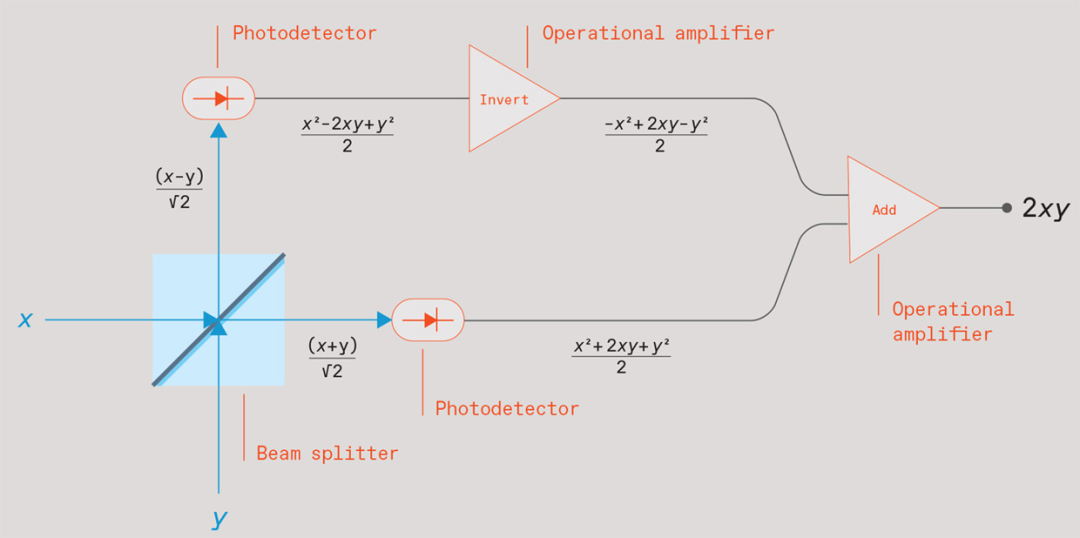图注：两束光线撞上一个光束分束器（蓝色方块），光束的强度与它们要相乘的数字 x 与 y 相当。离开分束器的光束照在光电探测器（椭圆形）上，提供与光强度平方成正比的电信号。将一个光电探测器信号调反，并将其与另一个信号相加，就会产生一个与两个输入的乘积成正比的信号。

所以，训练当今的大型神经网络通常会带来显著的环境问题。例如，2019 年的一项研究（“Energy and Policy Considerations for Deep Learning in NLP”）发现，训练一个特定的深度神经网络来进行自然语言处理所产生的二氧化碳排放量是汽车在整个生命周期内的驾驶所产生的二氧化碳排放量的五倍。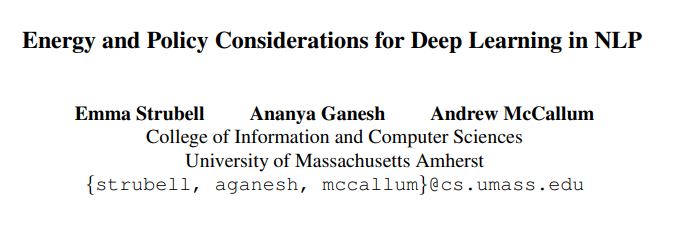论文地址：https://arxiv.org/pdf/1906.02243.pdf

3

光学应运而生

可以肯定的是，数字电子计算机的进步促进了深度学习的蓬勃发展，但这并不意味电子计算机是执行神经网络计算的唯一机器。数十年前，当数字计算机还相对原始时，一些工程师是使用模拟计算机来解决困难的计算。随着数字电子技术的进步，那些模拟计算机被淘汰了。

但现在也许是再次使用模拟计算机的好时机，尤其是当模拟计算可以通过光学的方式来完成时。

我们知道，光纤可以支持比电线高得多的数据速率。这也是为什么从 1970 年代后期开始，所有长途通信线路都采用光纤的原因。从那时起，光数据线路取代了铜线，已实现越来越短的跨度，一路延伸到数据中心的机架到机架通信。光数据通信速度更快，能耗也更低。光学计算也有同样的优势。

但数据传播与用数据进行计算有很大的区别。这也是模拟光学所遇到的障碍。传统的计算机是基于晶体管，而晶体管是高度非线性的电路元件——这意味着它们的输出不仅与输入成正比，至少在用于计算时是这样。非线性决定了晶体管的开关，使得它们可以被塑造成逻辑门电路。这种切换很容易用电子设备来完成，所以电子设备的非线性十分重要。但光子遵循的是麦克斯韦方程，是线性的，这就意味着光学设备的输出通常与其输入成正比。

在这里，一个诀窍是利用光学设备的线性来做深度学习最依赖的部分——线性代数。

为了解释如何做到这一点，我将在这里描述这样一个光子设备：当它与一些简单的模拟电子设备耦合时，它可以将两个矩阵相乘。这种乘法将一个矩阵的行与另一个矩阵的列组合在一起。更准确地说，它将这些行和列中的数对相乘，并将它们的乘积加在一起——也就是我之前描述的乘法和累加运算。我和我在 MIT 的同事在 2019 年发表了一篇文章（“Large-Scale Optical Neural Networks Based on Photoelectric Multiplication”），解释了为什么可以光子设备做到这一点。我们正在努力构建这样一个光学矩阵乘法器。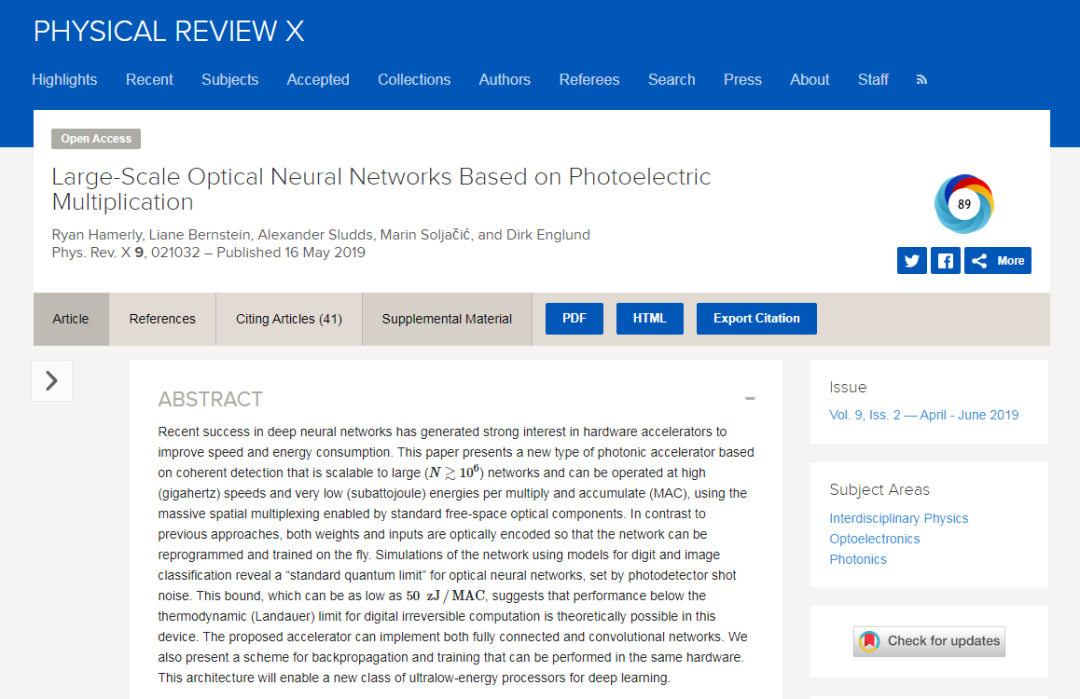论文地址：https://journals.aps.org/prx/abstract/10.1103/PhysRevX.9.021032

在这个光子设备中，最基础的计算单元是一个被称为“分束器”的光学元件。分束器的实际组装很复杂，但你可以把它想象成一个 45 度角的半镀银镜子。如果您从侧面向其发送一束光，分束器将允许一半光直接穿过它，而另一半则从有角度的镜子中反射，使其与入射光束成 90 度反弹。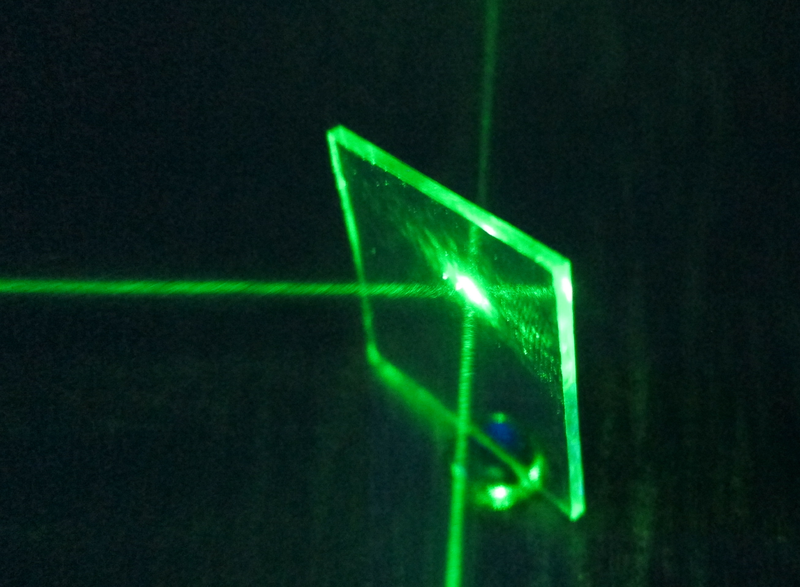图注：分束器

现在将第二束光垂直于第一束光照射到该分束器中，使其照射到成角度的镜子的另一侧。第二光束的一半将类似地以 90 度角透射和反射。两个输出光束将与第一个光束的两个输出成组合。所以这个分束器会有两个输入和两个输出。

要使用此设备进行矩阵乘法，你需要生成两个光束，且光束的电场强度与要相乘的两个数字成正比。我们将这些电场强度称为 x 和 y。将这两束光照射到分束器中，分束器会将这两束光合并在一起。这种特殊的分束器会产生两个输出，其电场值为 (x + y)/√2 和 (x − y)/√2。

除了分束器之外，这个模拟乘法器还需要两个简单的电子元件（两个光电探测器）来测量两个输出光束。不过，它们不测量这些光束的电场强度，而是测量光束的功率，该功率与其电场强度的平方成正比。

为什么这种联系很重要？要理解这一点，需要一些代数知识，但都是高中阶段的内容：当你对 (x + y)/√2 平方时，你会得到 (x2 + 2xy + y2)/2。当你平方 (x − y)/√2 时，你会得到 (x2 − 2xy + y2)/2。从前者中减去后者得到 2xy。

现在停下来思考这个简单数学的重要性。这意味着：如果你将一个数字编码为具有一定强度的光束，将另一个数字编码为另一种强度的光束，然后将它们通过这样的分束器发送，用光学探测器来测量两个输出，并在将它们相加之前抵消所产生的电信号之一，你就会得到一个与两个数字的乘积成正比的信号。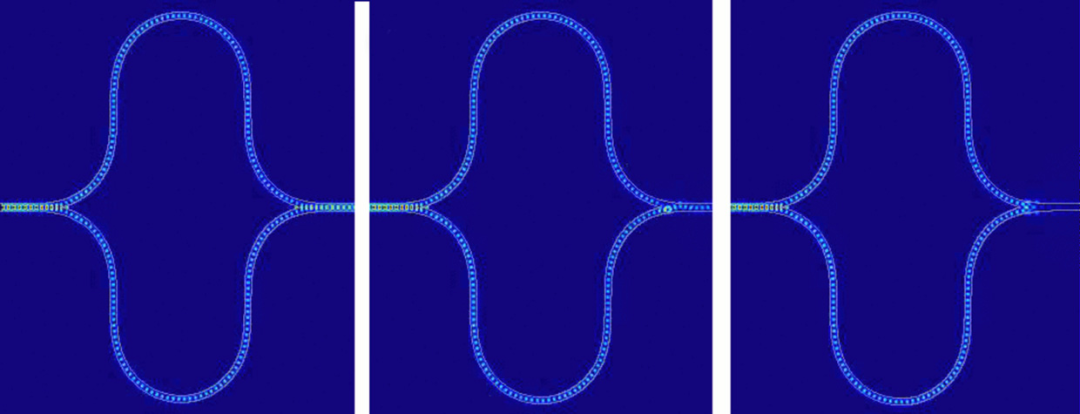图注：Lightmatter 的神经网络加速器中集成的 Mach-Zehnder 干涉仪模拟显示了三种不同的状态，其中在干涉仪的两个分支中传播的光经历了不同的相对相移。

我的描述听起来像是这些光束中的每一个光束都必须保持稳定。但事实上，你可以在两个输入光束中短暂地震动光并测量输出脉冲。更好的做法是，你可以将输出信号馈送到电容器中，只要震动持续，电容器就会积累电荷。然后，你可以在相同的时间段内再次脉冲输入，编码两个要相乘的新数字。它们的产品为电容器增加了一些电荷。你可以根据需要多次重复此过程，每一次都进行一次新的乘法累加运算。

以这种方式使用脉冲光可以让你飞速执行许多此类运算。其中，最耗能的部分是读取该电容器上的电压，这时需要一个模数转换器。但是你不必在每个脉冲后都这样做。你可以等到 N 个脉冲后结束后再统一读取。这意味着：该设备可以使用相同的能量来执行 N 次乘法累加运算，不管 N 是大是小。在这里，N 对应神经网络中每层的神经元数量，很容易达到数千个，所以用光子执行神经网络计算的能耗会很少。

有时候，你也可以在输入端节省能源，因为相同的值经常被用作多个神经元的输入。它不用多次将数字转换为光（每转换一次就会消耗一次能量），而是可以一次性转换所有，产生的光束可以被分成多个电流波段。通过这种方式，输入转换的能源成本可以在多次运算中分摊。

将一束光束分成多个波段需要透镜，但透镜很难安装在芯片上。因此，我们正在开发的以光学方式执行神经网络计算的设备很可能会是一种结合高集成光子芯片与单个光学元件的混合体。

4

其他实践案例

我在这里概述了我和我的同事一直在追求的策略，但从光学角度解决这个问题的方法不止一种。比如，另一个很有前景的方案是基于 Mach-Zehnder 干涉仪，它结合了两个分束器和两个全反射镜，也可以用光学方式运算矩阵乘法。两家有 MIT 学术背景的初创公司 Lightmatter 和 Lightelligence（沈亦晨创办） 正在开发基于 Mach-Zehnder 干涉仪的光学神经网络加速器。Lightmatter 已经制造出一个光学芯片原型，并有望在今年年底开始销售使用该芯片的光加速器板。

另一家使用光学进行计算的初创公司是 Optalysis。早在 1960 年代，光学计算的首批用途之一就是处理合成孔径雷达数据。但这有一个重大挑战，就是如何将傅立叶变换（一种数学运算）应用于要测量的数据上。当时的数字计算机一直在努力解决这些问题。即使是现在，将傅立叶变换应用于大规模数据也需要密集的计算。但是，傅立叶变换可以以光学的方式执行，只需要一个透镜（lens）——这也是多年来工程师处理合成孔径数据的方式。Optalysis 希望根据当前的需求改进这种方法，并广泛应用。

还有一家叫做 Luminous 的公司，源于普林斯顿大学，他们正致力于创建基于激光神经元的脉冲神经网络（spiking neural networks）。脉冲神经网络更像是模仿生物神经网络的运作方式，比如我们的大脑，能够使用低能量进行计算。Luminous 的硬件仍处于早期开发阶段，但结合脉冲和光学两种节能方法的潜力还是非常令人期待的！

5

光学的机遇与挑战

当然，目前仍有许多技术挑战需要克服。

一是要提高模拟光学计算的精度和动态范围，这方面还远不及数字电子设备所能达到的效果。这是因为这些光学处理器受到各种噪声源的影响，而且用于输入和输出数据的数模转换器和模数转换器精度有限。事实上，很难想象一个光学神经网络的运行精度超过 8 到 10 位。虽然存在 8 位电子深度学习硬件（比如 Google 的 TPU），但这个行业还需要更高的精度，尤其是用于神经网络训练时。

将光学元件集成到一块芯片上也很难。由于这些元件的尺寸为数十微米，它们无法像晶体管一样进行紧密封装，所以目标芯片的面积也会加大。2017年，来自 MIT 的团队（沈亦晨为一作）就针对这个问题发表了一篇工作（“Deep learning with coherent nanophotonic circuits”），谈到一种尺寸为 1.5 毫米的芯片。即使是最大的芯片也不会超过几平方厘米，这限制了可以用这种方式并行处理的矩阵的大小。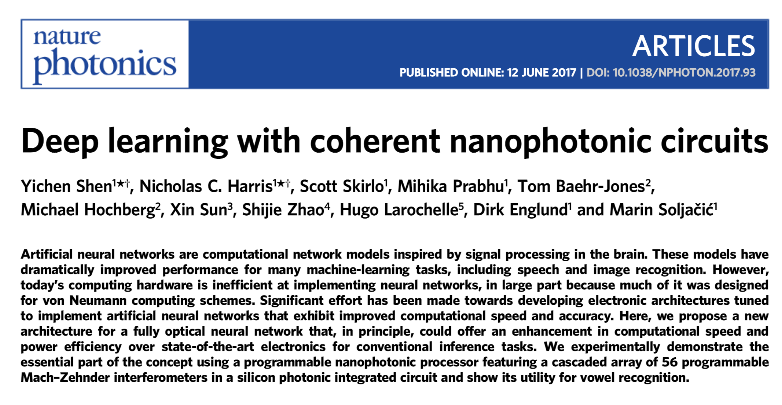论文地址：https://www.nature.com/articles/nphoton.2017.93.epdf

在计算机的架构方面，光学研究人员还有许多其他问题要解决。但可以肯定的是，至少在理论上，光学有希望将深度学习的发展加速几个数量级。

基于当前可用于各种组件（光调制器、检测器、放大器、模数转换器）的技术，我们有理由相信，神经网络计算的能源效率可以比当今的电子处理器提高 1,000 倍。如果用新兴的光学技术作出更激进的假设，神经网络计算的能源效率甚至可能提高一百万倍。而且，由于电子处理器的功率有限，这些能源效率的进步很可能会转化为相应的速度改进。

模拟光学计算中的许多概念已有数十年历史。有些概念的诞生甚至早于硅计算机。光学矩阵乘法、甚至光学神经网络的首次出现甚至可以追溯到1970年代。但当时这种方法并没有流行起来。但如今时代不同，光学计算的命运可能会有所改变，原因主要有三点：

• 首先，如今深度学习有真正的用途，而不仅仅是学术上的好奇；

• 其次，我们不能仅仅依靠摩尔定律来改进电子产品；

• 最后，我们有了前几代人没有的新技术：集成光子学。

这些因素表明，光神经网络将真正到来，而且，神经网络计算的未来可能是光子的。

原文链接：

推荐阅读

重磅！DLer-计算机视觉&Transformer群已成立！

大家好，这是计算机视觉&Transformer论文分享群里，群里会第一时间发布最新的Transformer前沿论文解读及交流分享会，主要设计方向有：图像分类、Transformer、目标检测、目标跟踪、点云与语义分割、GAN、超分辨率、视频超分、人脸检测与识别、动作行为与时空运动、模型压缩和量化剪枝、迁移学习、人体姿态估计等内容。

进群请备注：研究方向+学校/公司+昵称（如Transformer+上交+小明）???? 长按识别，邀请您进群！

展开全文神经网络 人工智能 深度学习 大数据
• 他用程序编写了一个数列生成，可以生成一个长度为N的数列，数列中的每个数都属于集合S。小C用这个生成生成了许多这样的数列。但是小C有一个问题需要你的帮助：给定整数x，求所有可以生成出的，且满足数列中所有...

【描述】
小C有一个集合S，里面的元素都是小于M的非负整数。他用程序编写了一个数列生成器，可以生成一个长度为N的数列，数列中的每个数都属于集合S。小C用这个生成器生成了许多这样的数列。但是小C有一个问题需要你的帮助：给定整数x，求所有可以生成出的，且满足数列中所有数的乘积mod M的值等于x的不同的数列的有多少个。小C认为，两个数列{Ai}和{Bi}不同，当且仅当至少存在一个整数i，满足Ai≠Bi。另外，小C认为这个问题的答案可能很大，因此他只需要你帮助他求出答案mod 1004535809的值就可以了。

【思路】

前置知识：
1.NTT应用拓展
这也是一道NTT好题。我们知道NTT和FFT可以快速计算如下形式的式子：
f [ k ] = ∑ i + j = k f [ i ] ∗ f [ j ] f[k]=\sum_{i+j=k}f[i]*f[j]
而在模数较小时，我们还可以支持快速计算如下形式的式子：
f [ k ] = ∑ i ∗ j = k f [ i ] ∗ f [ j ] f[k]=\sum_{i*j=k}f[i]*f[j]
怎么把乘法变为加法呢？取对数。所以设g为模数的一个原根，我们知道： g 0 , g 1 . . . . . . g m o d − 2 g^0,g^1......g^{mod-2} 是模意义下[1,mod-1]的一个排列。我们考虑把下标i的含义变为 g i g^i 就可以把乘法变为加法了。
2.找原根
我们先把mod-1质因数分解。对于mod-1的每个质因数p，我们验证一个数a是否在模意义下满足 a m o d − 1 p = 1 a^{\frac{mod-1}{p}}=1 即可。

下面进入正题。我们设生成函数 f ( x ) = ∑ a i ϵ S x log ⁡ g a i f(x)=\sum_{a_i\epsilon S}x^{\log_g a_i} ，设题目中要求的数列的积为need，那么我们要求的就是这个生成函数模意义下的n次方 x log ⁡ g n e e d x^{\log_g need} 的系数，注意这里的多项式乘法是循环卷积，而且是模mod-1意义下的循环卷积。当然这样的方法也可以理解为dp倍增优化，即设 f [ i ] [ j ] f[i][j] 是使用了i个数，乘积为j的方案数，倍增即可，这里倍增时也需要使用前置知识第一条优化为 O ( m log ⁡ m log ⁡ n ) O(m \log m\log n) ，否则 O ( m 2 log ⁡ n ) O(m^2\log n) 会超时。其实这两种方法本质是一样的，代码写起来都一个样子。
代码：

#include<bits/stdc++.h>
#include<tr1/unordered_map>
#define re register
using namespace std;
const int N=2e4+5;
const int mod=1004535809;
map<int,int>mp;
inline int red(){
int data=0;int w=1; char ch=0;
ch=getchar();
while(ch!='-' && (ch<'0' || ch>'9')) ch=getchar();
if(ch=='-') w=-1,ch=getchar();
while(ch>='0' && ch<='9') data=(data<<3)+(data<<1)+ch-'0',ch=getchar();
return data*w;
}
int n,m,a,b,c;
int f[N],lim=1,l=0,rev[N],g;
inline int add(const int&a,const int&b){return (a+b)>=mod?a+b-mod:a+b;}
inline int dec(const int&a,const int&b){return (a-b)<0?a-b+mod:a-b;}
inline int mul(const int&a,const int&b){return 1ll*a*b%mod;}
inline int ksm(int a,int b,const int&mod){
int ret=1;
while(b){if(b&1)ret=1ll*ret*a%mod;a=1ll*a*a%mod;b>>=1;}
return ret;
}
inline void pre(){lim=1;l=0;
while(lim<((m-1)<<1))lim<<=1,++l;
for(int re i=0;i<lim;++i)
rev[i]=(rev[i>>1]>>1)|((i&1)<<(l-1));
}
inline void ntt(int *f,int type){
for(int re i=0;i<lim;i++)if(i>rev[i])swap(f[i],f[rev[i]]);
int now=type==1?3:(mod+1)/3;
for(int re mid=1;mid<lim;mid<<=1){
int tmp=ksm(now,(mod-1)/(mid<<1),mod);
for(int re j=0;j<lim;j+=mid<<1){int w=1;
for(int re k=0;k<mid;k++,w=mul(w,tmp)){
int x=f[j+k],y=mul(w,f[j+k+mid]);
}
}
}
if(type==-1){
int inv=ksm(lim,mod-2,mod);
for(int re i=0;i<lim;++i)f[i]=mul(f[i],inv);
}
}
struct poly{
int ret;
friend inline poly operator*(poly a,poly b){
static poly c;memset(c.ret,0,sizeof(c.ret));
ntt(a.ret,1);ntt(b.ret,1);
for(int re i=0;i<lim;++i)c.ret[i]=mul(a.ret[i],b.ret[i]);
ntt(c.ret,-1);
for(int re i=m-1;i<lim;++i)c.ret[i]=0;
return c;
}
friend inline poly operator^(poly a,int b){
static poly c;memset(c.ret,0,sizeof(c.ret));c.ret=1;
for(;b;b>>=1,a=a*a)if(b&1)c=c*a;
return c;
}
}A;
int p[N],cnt=0;
inline void div(int m){
for(int re i=2;i*i<=m;++i){
if(m%i==0){
p[++cnt]=i;
while(m%i==0)m/=i;
}
}if(m>1)p[++cnt]=m;
}
inline void getg(){bool flag;div(m-1);
while(g=rand()%(m-1)+1){flag=0;
for(int re i=1;i<=cnt;i++){
if(ksm(g,(m-1)/p[i],m)==1){flag=1;break;}
}if(flag)continue;
else return;
}
}
int x;
int main(){
n=red();m=red();x=red();getg();pre();
for(int re p=g,i=1;i<m-1;(p*=g)%=m,++i)mp[p]=i;
for(int re i=red();i;--i)
if(a=red())A.ret[mp[a]]=1;
A=A^n;cout<<A.ret[mp[x]];
}

展开全文• 他用程序编写了一个数列生成，可以生成一个长度为N的数列，数列中的每个数都属于集合S。 小C用这个生成生成了许多这样的数列。但是小C有一个问题需要你的帮助：给定整数x，求所有可以生成出的，且满足数列中...
• 但是如果可能的话，我想匹配我的乘法算法的时间复杂度(目前是Toom-Cook)。我收集了一些线性时间算法，用于采用各种除法乘数逆的概念。这意味着从理论上讲，我可以以与乘法相同的时间复杂度来实现除法，因为无论如何...
• 他用程序编写了一个数列生成，可以生成一个长度为N的数列，数列中的每个数都属于集合S。小C用这个生成生成了许多这样的数列。但是小C有一个问题需要你的帮助： 给定整数x，求所有可以生成出的，且满足数列中...
• 3992: [SDOI2015]序列统计 Time Limit: 30 SecMemory Limit: 128 MBSubmit: 1888Solved: 898[Submit][Status][Discuss] ...他用程序编写了一个数列生成，可以生成一个长度为N的数 列，数列中的每个数都属于集...
• 多项式 之 快速傅里叶变换(FFT)/数论变换(NTT)/例题与常用套路【入门】 前置技能 对复数以及复平面有一定的了解 对数论要求了解：逆元，原根，中国剩余定理 对分治有充足的认识 对多项式有一定的认识，并会...
• CPU的功能和基本结构CPU的功能运算控制CPU的基本结构指令执行过程三个时间...的特点指令流水线基本概念指令流水的定义流水线的表示方法性能指标吞吐率加速比效率影响因素结构相关（资源冲突）数据相关（数据冲突微程序控制器 硬布线控制器 指令流水线
• 他用程序编写了一个数列生成，可以生成一个长度为N的数列，数列中的每个数都属于集合S。 小C用这个生成生成了许多这样的数列。但是小C有一个问题需要你的帮助：给定整数x，求所有可以生成出的，且满足数列中...
• 离，得到YUV 或YIQ 分量，然后用三个模／数转换对三个分量分别采样并进行 数字化，最后再转换成RGB 空间。 1、图像子采样  对彩色电视图像进行采样时，可以采用两种采样方法。一种是使用相同的采 样频率...
• 4、使用Karatsuba机制，提升长乘法的效率和精度 5、使用gcc/g++嵌入式汇编指令优化除法 6、其他 未来优化方向 1、使用SSE/SSE2、AVX指令集优化 2、使用并行计算优化 3、进制转换优化 4、增加乘数FFT缓存 2021年4月14...算法 c++
• 停课刷题总结

2019-05-11 09:28:00
CF576D Flights for Regular Customers 矩阵乘法 + Bitset优化 BZOJ 3879: SvT 虚树 + 后缀自动机 CF1073G Yet Another LCP Problem 后缀自动机 + 虚树 + 树形DP CF613D Kingdom and its Cities 虚树 + 树形DP ...
• ” 至于测量反馈部分，武居团队开发了一套系统，能在25微秒内执行100k x 100k矩阵和100k元元载体的乘法计算，即可满足5公里光纤腔的脉冲往返时间的要求。武居弘樹强调：“该系统所需FPGA芯片超过50个。如果未来还想...量子计算 量子计算机 量子力学 人工智能
• 数论变换（NTT）可用于多项式模的快速乘法 多项式模数和系数模数。 在这种情况下，变量using_ntt将设置为true。 但是，目前SEAL要求这是参数有效的情况。 因此，如果using_ntt为true，则parameters_set只能为true...SEAL
• 友链

2019-09-28 08:11:37
决策单调栈优化DP** 3.单峰极值 三分优化DP 4.四边形不等式 优化线性DP 优化区间DP **5.数据结构** **6.斜率 单调队列维护** 二分维护 平衡树维护 cdq分治维护 **7.矩阵乘法快速幂** **8.倍增 ST表**...
• 问题3：对拍中 Python 运行速度远远快于 C++ 解决方案：原来是对拍程序里的有个计时忘记累加时间了，改正后现问题已解决。 3. 设计的测试数据与测试结果 测试1为设计部分极端样例，观察程序是否符合预期。 输入...数据结构
• 上节课提出疑问：除了多周期CPU，还有什么技术可以提升速度？流水。这是我们计组的最后手段了。剩下的优化技术要交给计算机系统这门课了。编程语言 计算机组成
• 　离散对数NTT，循环卷积，求解原根，倍增优化dp。 　所谓循环卷积，就是在DFT回来统计答案的时候，除了加上对应位置$x$上的卷积结果，还要再加上$x+m-1$位置上的卷积结果。example： 序列统计 博弈论 ...
• 　离散对数NTT，循环卷积，求解原根，倍增优化dp。 　所谓循环卷积，就是在DFT回来统计答案的时候，除了加上对应位置$x$上的卷积结果，还要再加上$x+m-1$位置上的卷积结果。example： 序列统计 博弈论 　当前...
• 线段树优化的建图(单源最短路)(1)(2) DP的题目(30/130) 省选准备BLOG +1/-1的RMQ+LCA+笛卡尔树 复习内容 知识清单 密码hpxx【Orz】【请勿随便转载】 线性筛各种东西（素数，欧拉，莫比乌斯） ...知识点
• T2足足写了我3个小时之久……后面仍然想继续优化，未果。 然后开始尝试做T3题答。感觉就是模拟退火能做很多分的题啊，可惜我不会！最终只拿10分，有一个点比赛结束了还没调完，太惨了！ 只有清香鱼汉堡是我唯一...